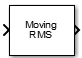Moving RMS

• Library:
• DSP System Toolbox / Statistics

•Description

The Moving RMS block computes the moving root mean square (RMS) of the input signal along each channel independently over time. The block uses either the sliding window method or the exponential weighting method to compute the moving RMS. In the sliding window method, a window of specified length moves over the data sample by sample, and the block computes the RMS over the data in the window. In the exponential weighting method, the block squares the data samples, multiplies them with a set of weighting factors, and sums the weighed data. The block then computes the RMS by taking the square root of the sum. For more details on these methods, see Algorithms.

Ports

Input

expand all

Data over which the block computes the moving RMS. The block accepts real-valued or complex-valued multichannel inputs, that is, m-by-n size inputs, where m ≥ 1 and n ≥ 1. The block also accepts variable-size inputs. During simulation, you can change the size of each input channel. However, the number of channels cannot change.

This port is unnamed until you set Method to Exponential weighting and select the Specify forgetting factor from input port parameter.

Data Types: single | double
Complex Number Support: Yes

The forgetting factor determines how much weight past data is given. A forgetting factor of 0.9 gives more weight to the older data than does a forgetting factor of 0.1. A forgetting factor of 1.0 indicates infinite memory – all previous samples are given an equal weight.

Dependencies

This port appears when you set Method to Exponential weighting and select the Specify forgetting factor from input port parameter.

Data Types: single | double

Output

expand all

The size of the moving RMS output matches the size of the input. The block uses either the sliding window method or the exponential weighting method to compute the moving RMS, as specified by the Method parameter. For more details, see Algorithms.

Data Types: single | double
Complex Number Support: Yes

Parameters

expand all

If a parameter is listed as tunable, then you can change its value during simulation.

• Sliding window — A window of length Window length moves over the input data along each channel. For every sample the window moves over, the block computes the RMS over the data in the window.

• Exponential weighting — The block multiplies the squares of the samples by a set of weighting factors. The magnitude of the weighting factors decreases exponentially as the age of the data increases, but the magnitude never reaches zero. To compute the RMS, the algorithm sums the weighted data and takes a square root of the sum.

When you select this check box, the length of the sliding window is equal to the value you specify in . When you clear this check box, the length of the sliding window is infinite. In this mode, the block computes the RMS of the current sample and all the previous samples in the channel.

Dependencies

This parameter appears when you set Method to Sliding window.

Specifies the length of the sliding window in samples.

Dependencies

This parameter appears when you set Method to Sliding window and select the check box.

When you select this check box, the forgetting factor is input through the lambda port. When you clear this check box, the forgetting factor is specified on the block dialog through the Forgetting factor parameter.

Dependencies

This parameter appears only when you set Method to Exponential weighting.

The forgetting factor determines how much weight past data is given. A forgetting factor of 0.9 gives more weight to the older data than does a forgetting factor of 0.1. A forgetting factor of 1.0 indicates infinite memory – all previous samples are given an equal weight.

Tunable: Yes

Dependencies

This parameter appears when you set Method to Exponential weighting and clear the Specify forgetting factor from input port check box.

• Code generation

Simulate model using generated C code. The first time you run a simulation, Simulink® generates C code for the block. The C code is reused for subsequent simulations, as long as the model does not change. This option requires additional startup time but provides faster simulation speed than Interpreted execution.

• Interpreted execution

Simulate model using the MATLAB®  interpreter. This option shortens startup time but has slower simulation speed than Code generation.

Block Characteristics

 Data Types double | single Multidimensional Signals No Variable-Size Signals Yes

expand all

Extended Capabilities

C/C++ Code GenerationGenerate C and C++ code using Simulink® Coder™.

Introduced in R2016b

DSP System Toolbox DocumentationGet trial now